Introduction to Data Analysis

# 9.2. Smoothing

# Load packages.
"XML")
packages <- lapply(packages, FUN = function(x) {
if (!require(x, character.only = TRUE)) {
install.packages(x)
library(x, character.only = TRUE)
}
})


This example is based on online ratings of TV shows. It was first coded at Diffuse Prior, and Andy at Premier Soccer Stats have even coded it as an interactive graph with Shiny. We'll start by scraping the data from GEOS. The series under scrutiny is Aaron Sorkin's The West Wing.

file = "data/geos.tww.csv"
if (!file.exists(file)) {
# Parse HTML content.
html <- htmlParse("http://www.geos.tv/index.php/list?sid=179&collection=all")
# Select table on id.
html <- xpathApply(html, "//table[@id='collectionTable']")[]
# Convert to dataset.
# First data rows.
# Save local copy.
write.csv(data[, -3], file, row.names = FALSE)
}
data <- read.csv(file, stringsAsFactors = FALSE)
# Check result.
str(data)

'data.frame':   156 obs. of  5 variables:
$X : int 1 2 3 4 5 6 7 8 9 10 ...$ Title: chr  "Pilot" "Post Hoc, Ergo Propter Hoc" "A Proportional Response" "Five Votes Down" ...
$Rank : int 48 98 36 72 71 41 90 89 39 13 ...$ Mean : chr  "7.40 Â± 2.66" "6.89 Â± 2.62" "7.56 Â± 2.82" "7.13 Â± 2.79" ...
$Count: int 21 19 18 18 18 19 18 18 17 18 ...  Mean is the average rating of each episode, so we have a parameter, and Count is the number of votes on each episode, so we have a sample size. Using the equation for the standard error, $$SE = \frac{SD}{\sqrt{N}}$$, we will calculate the “margin of error” of each rating. Note that the distribution of the ratings is not normal due to a few episodes having received very high ratings. # Convert means from text. data$mu <- as.numeric(substr(data$Mean, 0, 4)) # Compute standard errors. data$se <- with(data, sd(mu)/sqrt(as.numeric(Count)))


The last step that we take with the data is to add the season number to be able to discriminate them visually later on. Each season of The West Wing has 22 episodes, except for two special cases (the final season has 23 episodes and one show is a film special). We use the remainder of a division by 22 to compute seasons, fix the special cases, and factor the variable for plotting purposes.

# Compute season.
data$season <- 1 + (data$X - 1)%/%22
# Fix special cases.
data$season[which(data$season > 7)] <- c(7, NA)
# Factor variable.
data$season <- factor(data$season)


The final plot uses 95% and 99% confidence intervals to visualize (some of the) uncertainty.

g = qplot(data = data, x = X, y = mu, colour = season, geom = "point") +
geom_linerange(aes(ymin = mu - 1.96*se, ymax = mu + 1.96*se), alpha = .5) +
geom_linerange(aes(ymin = mu - 2.58*se, ymax = mu + 2.58*se), alpha = .5) +
scale_colour_brewer("Season", palette = "Set1") +
scale_x_continuous(breaks = seq(1, 156, 22)) +
theme(legend.position = "top") +
labs(y = "Mean rating", x = "Episode")
g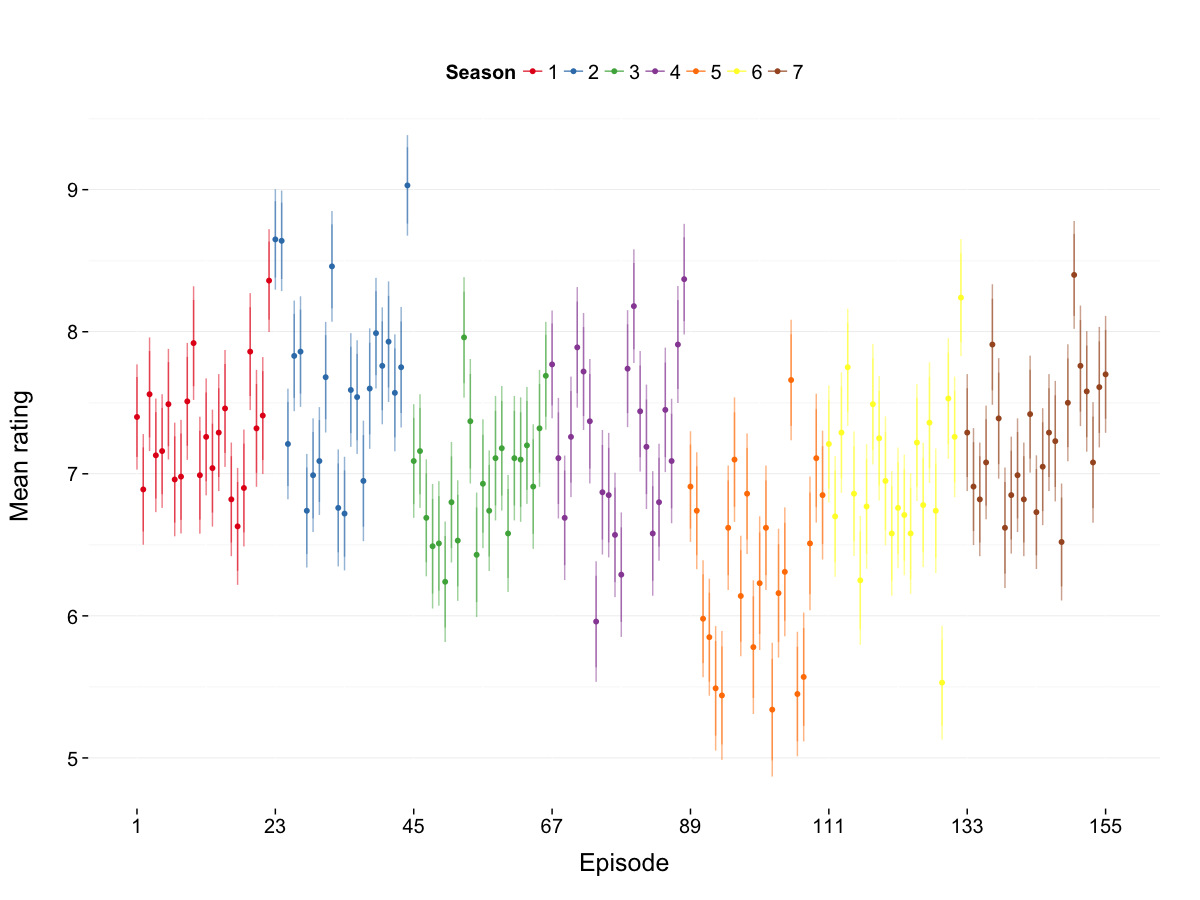The plot would be more useful with average ratings per season, which are easy to retrieve with the ddply() function. The minimum and maximum episode numbers are also computed to be able to plot horizontal segments for each season. Since we saved the previous plot as a ggplot2 object, adding the lines only requires to code the extra graphical element and add it on top of the saved elements.

# Compute season means.
means <- ddply(data, .(season), summarise,
mean = mean(mu),
xmin = min(X),
xmax = max(X))
g + geom_segment(data = means,
aes(x = xmin, xend = xmax, y = mean, yend = mean))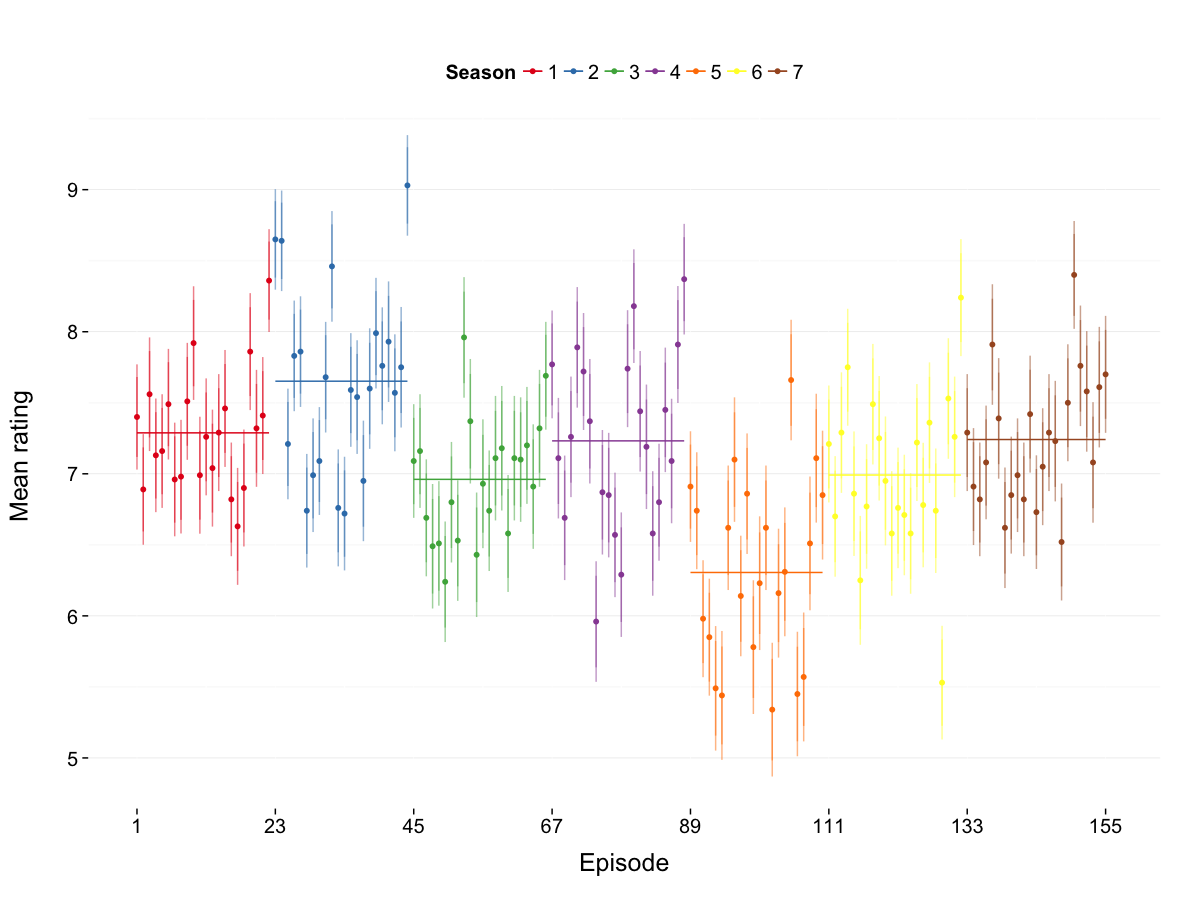## Changepoints

If your aim is to find abrupt variations in the series, use a changepoint algorithm, like the one below from the changepoint library.

# Compute changepoints with PELT algorithm.
cpt <- cpt.mean(data$mu, method = 'PELT') # Extract results. seg <- data.frame(cpt = attr(cpt, "param.est")) seg$xmax <- attr(cpt, "cpts")
seg$xmin <- c(0, seg$xmax[-length(seg$xmax)]) # Plot. g + geom_segment(data = seg, aes(x = xmin, xend = xmax, y = mean, yend = mean), color = "black")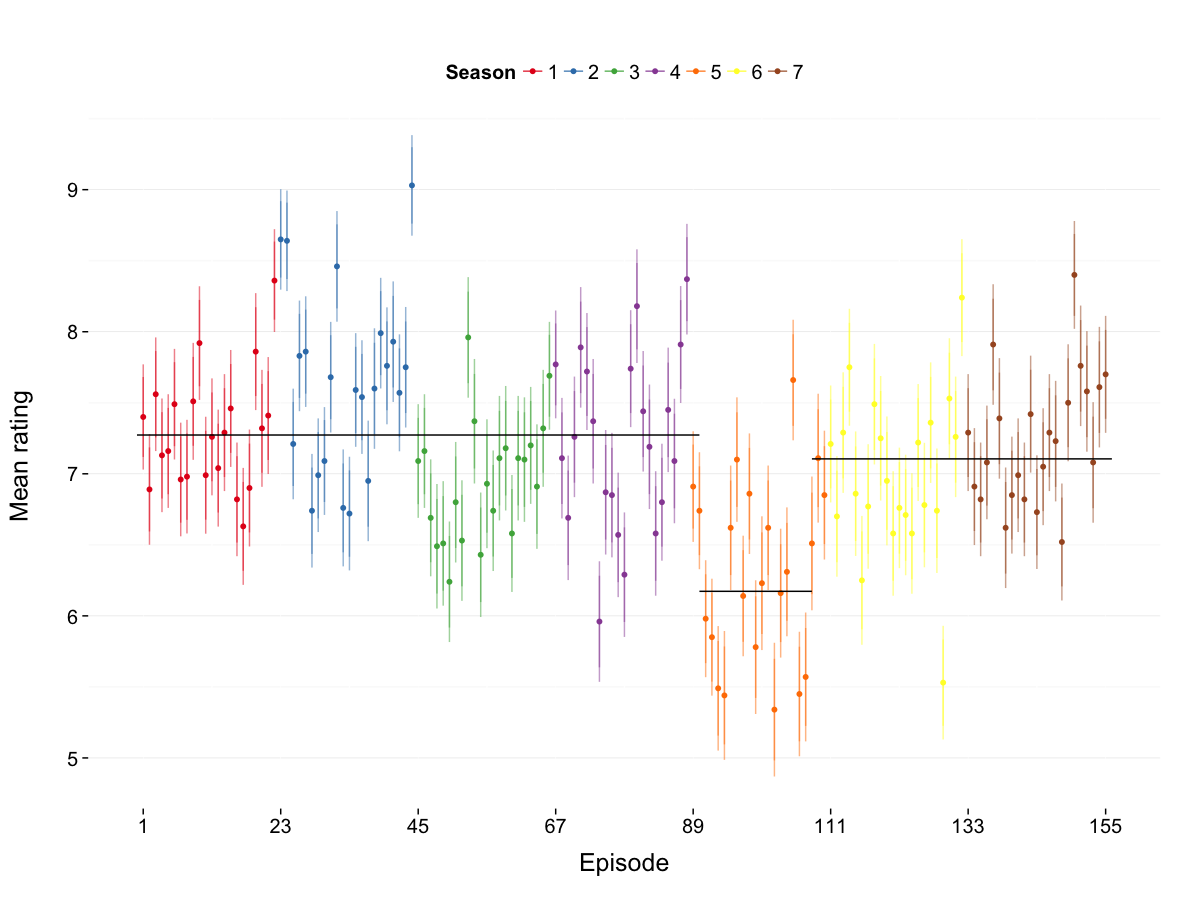By the way, if you find a way to predict this kind of data, let Netflix know and submit your work early. ## Smoothing: LOESS and cubic splines Let's now smooth the series. The base plots will all use the same data, to which we are going to overlay a trendline computed through different methods. # General plot function. g = qplot(data = data, x = X, y = mu, alpha = I(0.5), geom = "line") + scale_x_continuous(breaks = seq(1, 156, 22)) + labs(y = "Mean rating", x = "Episode")  The simplest ways to smooth the data is to use local polynomials, which we apply to the scores of each season, and then to their detrended values. The more complex smoothing method uses splines and is inspired by Kieran Healy's code for state-by-state homicide trends in the USA and other OECD countries. It is used only in the last plot. # LOESS smoother for each season. g + geom_smooth(se = FALSE) + geom_hline(y = mean(data$mu), linetype = "dashed") +
aes(colour = season)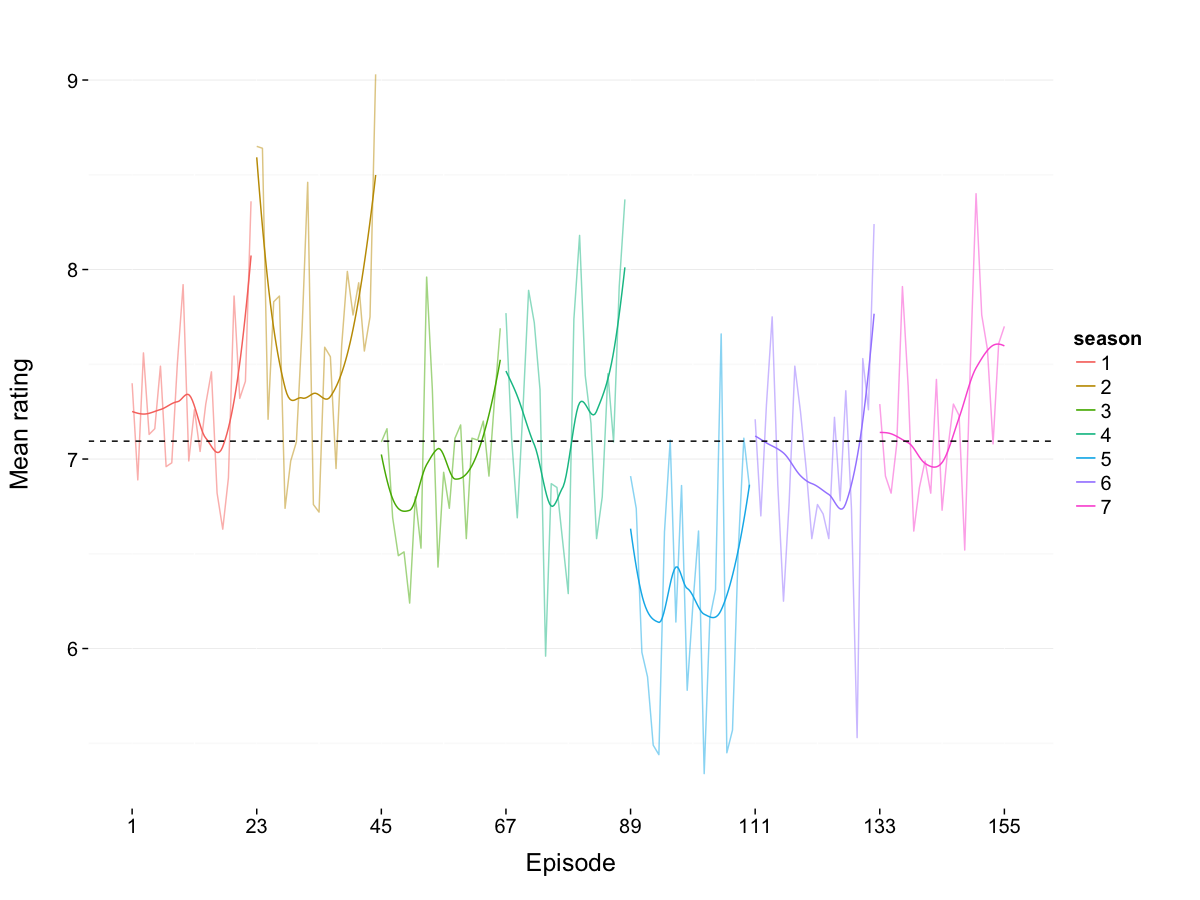# LOESS smoother for the detrended values.
g +
geom_smooth(se = FALSE) +
geom_hline(aes(y = mean(diff(mu))), linetype = "dashed") +
aes(colour = season, y = c(0, diff(mu)))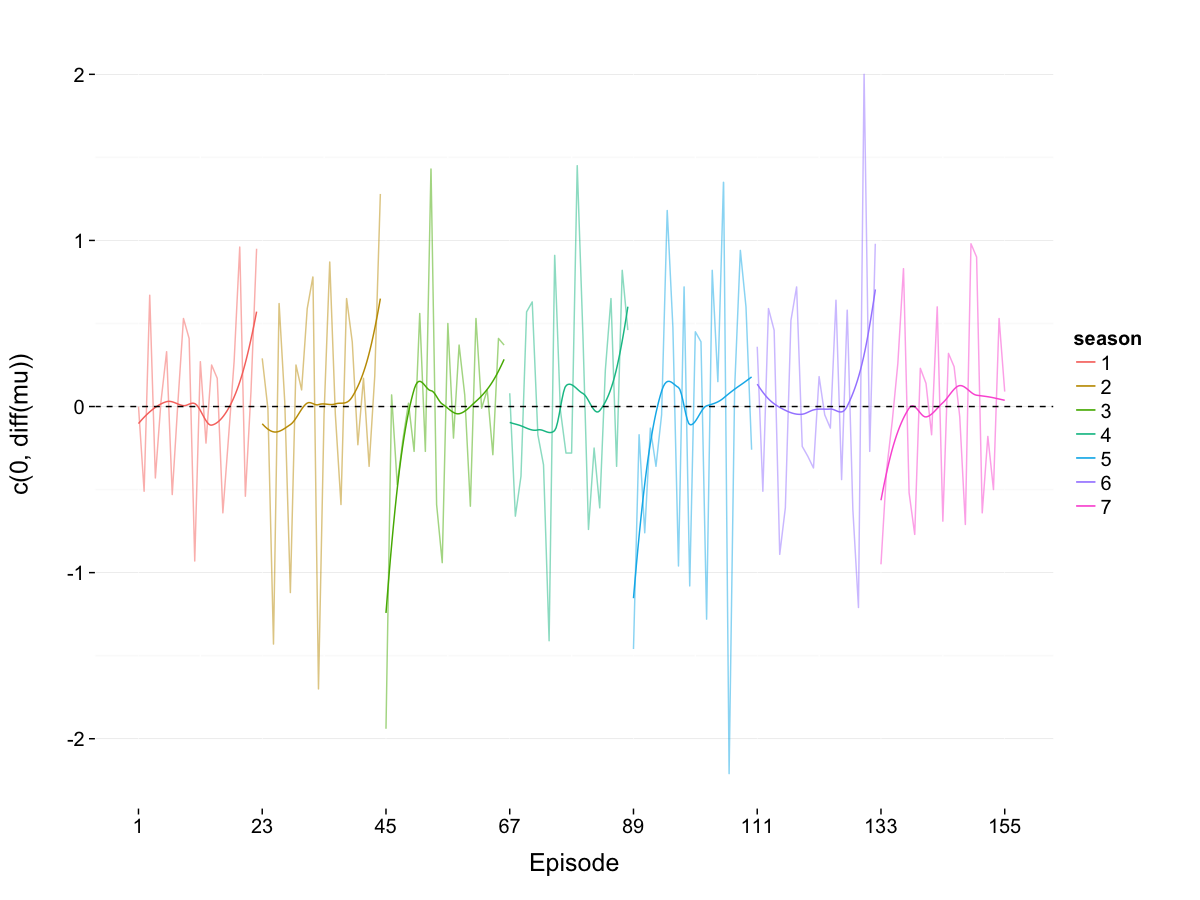# Cubic splines for the full series.
g +
geom_smooth(method = "rlm", se = FALSE, formula = y ~ ns(x, 8)) +
geom_hline(y = mean(data$mu), linetype = "dashed")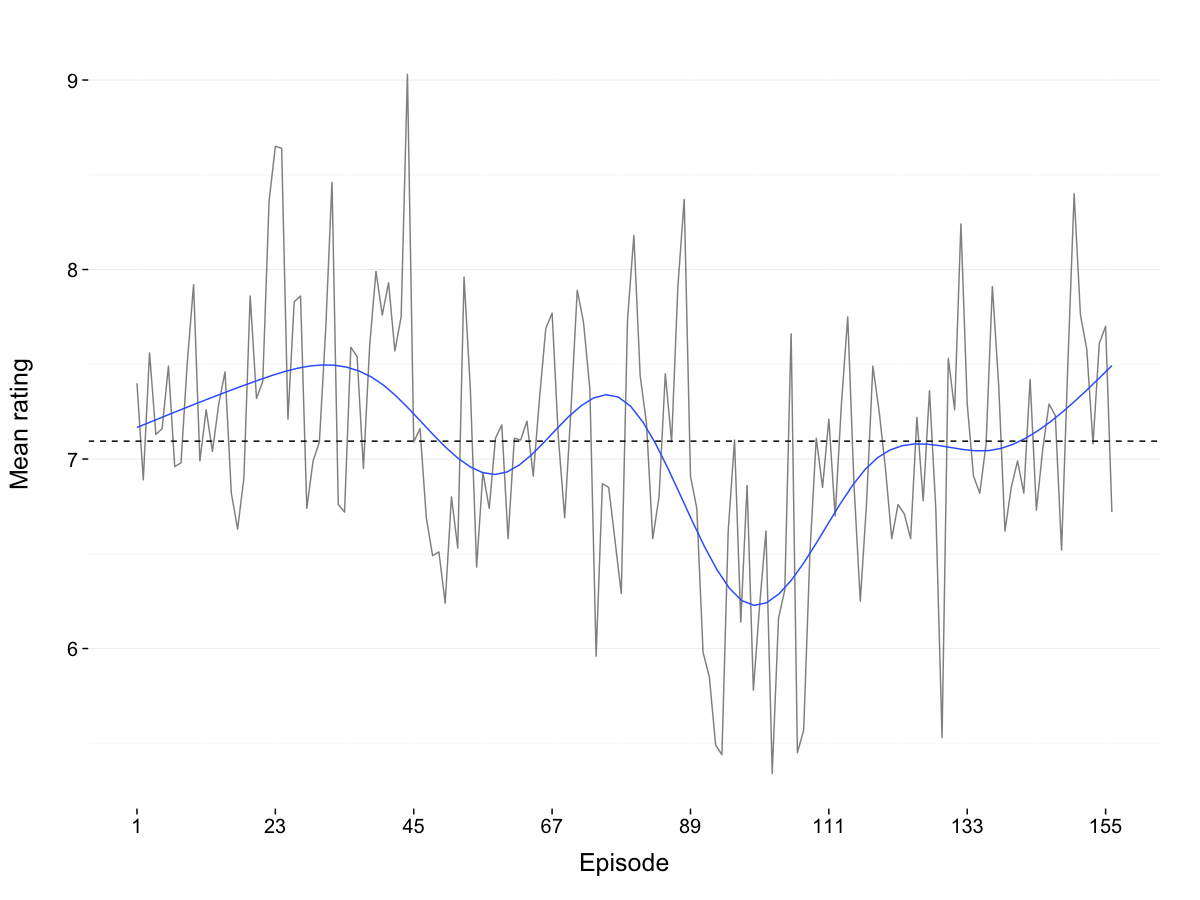The information provided by the cubic splines is almost identical to what we learnt from the changepoints: the series has three periods, due to one drop in audience ratings. # Cubic splines and changepoints. g + geom_smooth(method = "rlm", se = FALSE, formula = y ~ ns(x, 8)) + geom_hline(y = mean(data$mu), linetype = "dashed") +
geom_segment(data = seg,
aes(x = xmin, xend = xmax, y = mean, yend = mean),
color = "black")